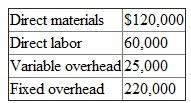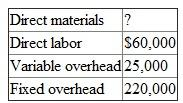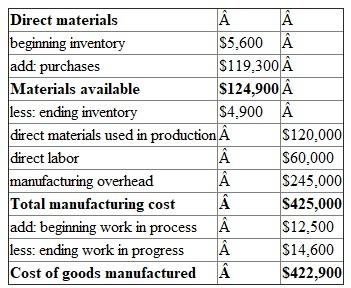# Quiz 2: Basic Cost Management Concepts

Pietro frozen foods predict that 50,000 frozen pizzas will be produced for the next year. Given:1.Unit prime cost is calculated as:Hence, the prime cost per unit is 2.Unit conversion cost is calculated as:Hence, the conversion cost per unit is 3.The total variable cost per unit is calculated as:Hence, the total variable cost per unit is 4.The total product cost per unit is calculated as:Hence, the total product cost per unit is 5.Since the variable costs increases by 10% the total direct materials, total direct labor, and total variable overhead would also increase by 10%. Total fixed overhead would remain the same. Unit prime cost will increase by 10 percent since both direct materials and direct labor are strictly variable, and 10 percent more units would require 10 percent more variable cost. The unit conversion cost would increase by less than 10 per-cent because of the presence of fixed costs. New unit product cost :Pietro frozen foods predict that 50,000 frozen pizzas will be produced for the next year Given:Pietro expects to purchase \$119,300 of direct materials. From the information given for the inventories the following calculations are made: 1.The statement of cost of goods manufactured in good form is as follows:2.If the ending inventory of direct materials will be increased by \$2,000 then the direct materials used in the manufacturing process will be decreased by \$2,000. Hence, the total manufacturing costs will also be decreased by \$2,000 and the cost of goods manufactured will also reduce by \$2000.